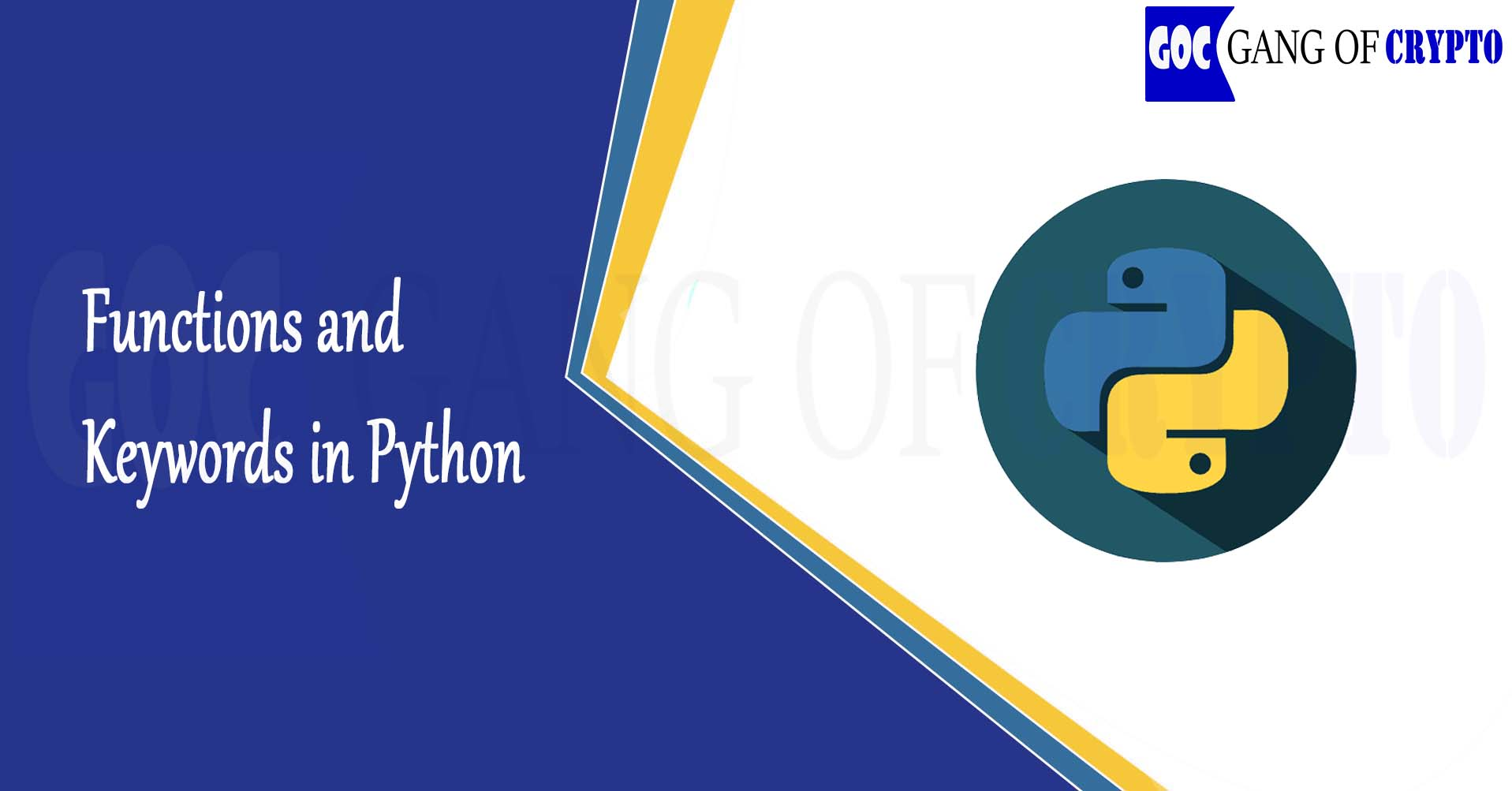# Functions and Keywords in Python

## Functions and Keywords in Python

Functions are pieces of code that perform a unit of work. In the examples, we have seen so far we’ve only encountered the print( ) function, which prints a message to the screen. We’ll learn about a lot of other functions in later lessons but, if you are too curious to wait until then, you can discover all the functions available here.
Also, read how python started here

Keywords are reserved words that are used to construct instructions. We briefly encountered for and in our first Python example, and we’ll use a bunch of other keywords as we go through the course. Functions and keywords in Python are building blocks of code. For reference, these are all the reserved keywords:

## Arithmetic Operators:

Python can operate with numbers using the visual mathematical operators, and some special operators, too. These are all of them ( we’ll explore the last two in later videos).

• a + b = adds a and b
• a – b = subtracts b from a
• a * b = multiplies a and b
• a / b = divides a by b
• a ** b = elevates a to the power of b.  For non-integer values of b, this becomes a root ( i.e. a**(1/2) the square root of a )
• a // b = the integer part of the integer division of a by b

a % b = the remainder part of the integer division of a by b

Example: Output a message that says “Programming with Python is fun!” to the screen.

``print("Programming with Python is fun!") ``